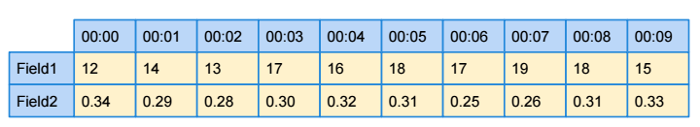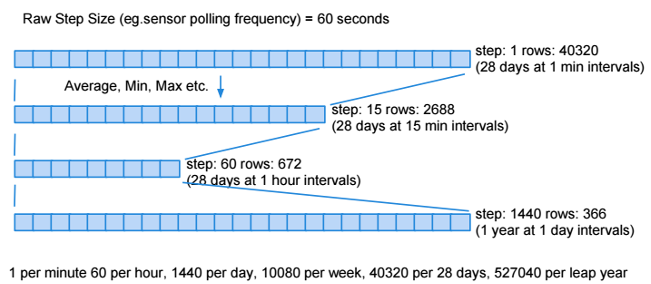Introduction
Features
Integration

## Introduction

Elixir Tempo is a database for storing fast changing time-series data. Examples of such data where values change over time include:

• Continuous Throughput (Counter)
• Rate of Change (Derive)

Data is stored in a Round Robin Database (RRD).

Figure 5.1, “Round Robin Database” shows an example of the RRD.

Figure 5.1. Round Robin DatabaseA field in an RRD is defined by the size of a step (here 1 minute) and the number of steps in a row (here 10).

This RRD only holds 10 minutes of data; the 11th minute will wrap-around and overwrite the first slot.

You can consolidate data as shown in example: Figure 5.2, “Consolidate Data”:

Figure 5.2. Consolidate Data